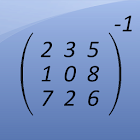Matrix Inversion Calculator

• 검색 유형

모든 Android 애플리케이션 카테고리

모든 Android 게임 카테고리# Matrix Inversion Calculator

by: 507 7.8

7.8 사용자
평가 등급

## 스크린샷

설명

This free app is a math calculator, which is able to calculate the invertible of a matrix.

The following matrices can be inverted:
- 2x2 matrices
- 3x3 matrices
- 4x4 matrices

Best math tool for school and college! If you are a student, it will helps you to learn linear algebra!
Note: The inverse of a matrix A is the matrix B, with AxB=I where I is the identity matrix and the multiplication used is ordinary matrix multiplication. A matrix is a nonsingular matrix if it is an invertible matrix, and the determinant of this matrix does not equal 0.

: 507 리뷰

"우수"

7.8